# Working with Functions

The previous sections explained how to enter a formula once, using relative cell referencing, and copy that formula to other cells . Although you only have to type the formula one time, this kind of totaling formula is tedious to type and introduces greater chance for error:

` =B6+B7+B8+B9+B10+B11+B12+B13+B14+B15+B16+B17 `

Fortunately, Microsoft includes several built-in functions that perform many common mathematical calculations. Instead of writing a formula to sum a row or column of values, for example, use the Sum() function.

Function names always end with parentheses, such as Average() . A function accepts zero or more arguments , and an argument is a value that appears inside the parentheses that the function uses in some way. Always separate function arguments with commas. If a function contains only a single argument, you do not use a comma inside the parentheses. Functions generally manipulate data ( numbers or text), and the arguments inside the parentheses supply the data to the function. The Average() function, for example, computes an average of whatever list of values you pass in the argument. Therefore, all the following compute an average from the argument list:

` =Average(18, 65, 299, \$R, 10, -2, 102) =Average(SalesTotals) =Average(D4:D14) `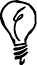When you begin to enter a formula, ScreenTips pop up after you type the formula's name to help guide you through the formula's required contents.

As with many functions, Average() accepts as many arguments as needed to do its job. The first Average() function computes the average of seven values, one of which is an absolute cell reference. The second Average() function computes the average of a range named SalesTotals . No matter how many cells compose the range SalesTotals , Average() computes the average. The last Average() function computes the average of the values in the range D4 through D14 (a columnar list).

The following formula computes the average of seven arguments, one of which ( F14 ) is a cell reference and one of which ( \$R\$5 ) is an absolute cell reference:

` =Average(18, F14, 299, \$R, 10, -2, 102) `

The Sum() function is perhaps the most common function because you so often total columns and rows. In the preceding section, you entered a long formula to add the values in a column. Instead of adding each cell to total the range B6:B17, you could more easily enter the following function:

` =Sum(B6:B17) `

If you copy this Sum() function to the other cells at the bottom of the yearly projections, the total appears at the bottom of those columns.When you insert rows within the Sum() range, Excel updates the range inside the Sum() function to include the new values.

#### To Do: Use AutoSum for Efficiency

Before looking at a table of common functions that you can use in your worksheets, consider that one of the activities you'll do the most is adding numbers in formulas. You'll need to add to compute totals, count items, and compute days between activities. Excel helps you add values by analyzing ranges that you select and automatically inserting a Sum() function if needed, thus computing the total. Here's how to do that:

1. Select the range that you want to sum. If you want to sum the months over the projected years for this hour 's sample worksheets, for example, select the row with the January label, as shown in Figure 7.7.

##### Figure 7.7. Getting ready to request a sum.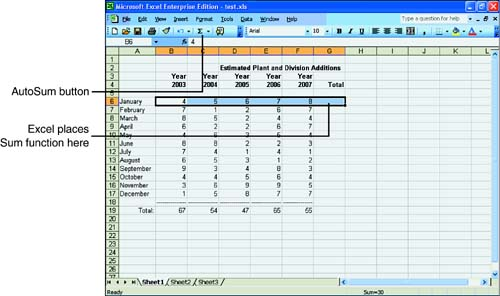2. Click the AutoSum toolbar button. If you don't see the AutoSum button on your toolbar, click the Toolbar Options button to locate it. Excel guesses that you want to sum the selected row and inserts the Sum() function in the cell to the right of the row.

3. Make any edits to the summed value if Excel included too many or not enough cells. You can click the cell and press F2 to edit the sum. Usually, no edits are required.

After Excel generates the Sum() function, you can copy the cell down the rest of the column to add the monthly totals. However, can you see another way to perform the same monthly totals with one selection? Select the entire set of monthly values with one extra blank column at the right (the range B6:G17). Excel sees the blank column and fills it in with each row's sum when you click AutoSum. You now can select the new column of totals and let AutoSum compute them. Figure 7.8 shows the result of the new sums after you add underlines and a title to the row.

##### Figure 7.8. AutoSum in action.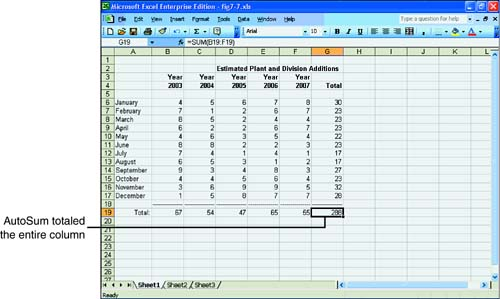#### Common Functions

Functions improve your accuracy. If you want to average three cell values, for example, you might type something such as

` =C2 + C4 + C6 / 3 `

This formula does not compute an average! Remember that the operator hierarchy forces the division calculation first. If you use the Average() function, as shown next , you don't have to worry as much about the calculation's hierarchy.

` =Average(C2, C4, C6) `Another advantage of using functions is that you can modify them more easily than long calculations. If you want to include another cell value into the previous average, for example, you only need to add the extra cell to Average() ; if you use a formula, you must remember to change the 3 to 4.

Table 7.2 describes common Excel built-in functions that you find a lot of uses for as you create worksheets. Remember to start every formula with an equal sign and to add your arguments to the parentheses, and you are set!

##### Table 7.2. Common Excel Functions

Function Name

Description

Abs()

Computes the absolute value of its cell argument. (Good for distance- and age-difference calculations.)

Average()

Computes the average of its arguments.

Count()

Returns the number of numerical arguments in the argument list. (Useful if you use a range name for the argument list.)

CountBlank()

Returns the number of blank cells, if any exist, in the argument range. (Useful if you use a range name for the argument list.)

Max()

Returns the highest (maximum) value in the argument list. (Useful if you use a range name for the argument list and you need to pick out the highest value.)

Min()

Returns the lowest (minimum) value in the argument list. (Useful if you use a range name for the argument list and you need to pick out the lowest value.)

Pi()

Computes the value of mathematical pi (requires no arguments) for use in math calculations.

Product()

Computes the product (multiplicative result) of the argument range.

Roman()

Converts its cell value to a Roman numeral.

Sqrt()

Computes the square root of the cell argument.

Stdev()

Computes the argument list's standard deviation.

Sum()

Computes the sum of its arguments.

Today()

Returns today's date (requires no arguments).

Var()

Computes a list's sample variance.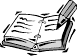Excel supports many functions, including complex mathematical, date, time, financial, and engineering functions. Click F1 or type a question in the Ask a Question box for Help to supply more details on all the functions you can use.

Some of the functions require more arguments than a simple cell or range. Excel contains many financial functions, for example, that compute loan values and investment rates of return. If you want to use one of the more advanced functions, click on an empty cell and select Insert, Function or click the Insert Function button to display the Insert Function dialog box, as shown in Figure 7.9.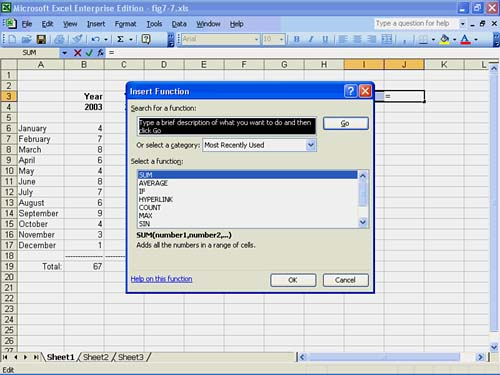You can select from a category of functions in the drop-down list box or describe what you want to do at the top of the dialog box and let Excel locate a function that might work. When you decide on a function (you can simply scroll the list of function names at the bottom of the dialog box and select one), Excel displays an additional dialog box with text box areas for each of the function arguments, such as the one shown in Figure 7.10. As you continue entering arguments that the function requires, Excel builds the function in the cell for you. As you get more proficient, you no longer need the help of the Insert Function dialog box as often.

##### Figure 7.10. You can quickly enter arguments in the Function Arguments dialog box.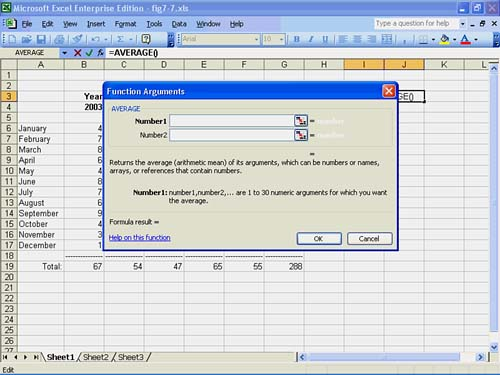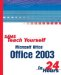Sams Teach Yourself Microsoft Office 2003 in 24 Hours
ISBN: 0672325535
EAN: 2147483647
Year: 2003
Pages: 272
Authors: Greg Perry